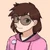## FANDOM

25,892 Pages# Amelia Lonelyheart

## aka Amelia

• I live in North Carolina, sadly.
• My occupation is writer, aspiring teacher
• Bio Hay
•## DC Comics: Mon-El moves a White-Dwarf Star

June 25, 2020 by Amelia Lonelyheart

Not really a calc, more of a reference.

Moves a White Dwarf at FTL speeds, so I'm using the GBE of it.

Feat here

Reference sheet for the smallest White dwarf

Mass: 0.17 MO
Diameter: 0.08 Solar diameter

g = G * M / r^2

G = 6.67 * 10^-11
M = 3.3813e+29 kg
R = 114,678 km

6.67 * 10^-11 * 3.3813e+29 / 114678000^2 = 1714.94153178

I'm unsure how to use the GBE formula, so please forgive me if I mess up. Putting the values in Wolfram Alpha I get 3.992×10^40 J or 9.54 Tenatons

I probably messed this up.

•## DC Comics: Superboy pushes the Earth

June 25, 2020 by Amelia Lonelyheart

Calc requested by User:SuperAPM and User:Zark2099. Feat happens in Young Justice #1,000,000. Superboy pushes the Planet out of orbit. The feat is probably more impressive then what I'm making it out to be, but theirs no real way to scale it.

Feat here

Earth orbits at 29.78 km/s, or 29,780 m/s

5.972e+24 * 0.5 * 29,780^2 = 2.6481293e+33 J

632.91 Zettatons

•## Marvel: Drax, the Destroyer of Worlds

June 24, 2020 by Amelia Lonelyheart

Calcs requested by Loser Man.

AFAIK, this planet has no name, much less a description, so I'll assume Earth-like conditions.

Feat here

Planet is 86 px, the furthest, major piece of 26 px away. Circle is admittedly a bit arbitrary, but it's roughly where the curves are, so it seems mostly fine. 🤷‍♀️

Earth = 12,472 km

86 / 26 = 3.307

12,472 / 3.307 = 3,771 km

Timeframe is a scream, so...10 seconds? 30 seconds as a low end.

3,771 km / 10 seconds = 377,100 m/s

3,771 km / 30 seconds = 125,700 m/s

Mass of Earth = 5.972 × 10^24 kg

5.972 × 10^24 * 0.5 * 377,100^2 = 4.2462237e+35 J = 101 Yottatons

5.972 × 10^24 * 0.5 * 125,700^2 = 4.7180263e+34 J = 11 Yottatons

Not sure what issue this is from. scan

Scaling here

Rough diameter of the planetoid is 201 px. Size of the frame is 639 …

•## Marvel Comics: Golden Age Cap stops a car

June 16, 2020 by Amelia Lonelyheart

Self-explanitory.

1940s Sedans weighed 4,300 pounds, or 1,950 kg

I surprisingly couldn't find a figure on the speed of a 1940 Sedan, but I found this document saying that the average speed of post-war cars were 40 MPH. Might be a bit inflated, but if anyone else can find a better figure, please let me know!

1,950 * 0.5 * 17.8816^2 = 311,757.8 J

311.7 KJ

So Class 1 LS and Wall level AP. Not bad.

Edit: Didn't notice Bulky was there too.

1,950 / 2 = 975 kg

311.7 / 2 = 155.85 KJ

Taken from his comment:

The force they applied here (for lifting strength) isn’t just the weight of the car, but the force of its deceleration. To get that we can take the energy it had and divide that by the distance it decelerated over. Then we can divide by gravitational acceleration…

•## The God of High School: Stigama Terra

June 9, 2020 by Amelia Lonelyheart

Calc requested by User:Sir Ovens.

Not a great circle, but it matches the top and east part of the globe well enough. I was considering scaling off the island, but I couldn't find a real-life counterpart. It seems decently big, so I might use Jeju or something, if the globe is considered unreliable.

Globe = 1,360 px = 12,742 km

Height is 78 px

1,360 / 78 = 17.43

12,742 / 17.43 = 731 km

Width is 87 px

1,360 / 87 = 15.63

12,742 / 15.63 = 815.227 km

Length is 527 px

1,360 / 527 = 2.58

12,742 / 2.58 = 4,938.75 km

For the small arm, the width is 41 px

1,360 / 41 = 33.17

12,742 / 33.17 = 384.14 km

And the length is 176 px

1,360 / 176 = 7.72

12,742 / 7.72 = 1,650.51 km

LWH

4,938.75 * 815.227 * 731 = 2,943,153,915.11 km^3

1,650.51 * 384.14 * 731 = 463,473,672.233 km^3

Clouds seem thicc so…